## Tuesday, August 31, 2010

### 490

490 = 2 x 5 x 72.

490 has a representation as a sum of two squares: 490 = 72 + 212.

490 is the number of integer partitions of 19.

490 is the sum of six positive fifth powers: 490 = 15 + 15 + 15 + 15 + 35 + 35.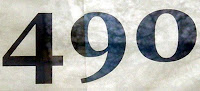The USS Leader (MSO 490) was a minesweeper launched in 1954.

## Monday, August 30, 2010

### 811

811 is a prime number.

809 and 811 form a twin prime pair.

811 is the smallest prime that is a sum of primes beginning and ending with the digit 7: 811 = 7 + 7 + 797.

811 is the sum of five consecutive primes: 811 = 151 + 157 + 163 + 167 + 173.

811 is a prime of the form 4n + 3 that is the sum of two consecutive composite numbers: 811 = 4(202) + 3 = 405 + 406.In the United States, the telephone number 811 has traditionally been a direct line to the business off of the telephone company providing service on the line.

## Friday, August 27, 2010

### 393

393 = 3 x 131.

1000393 - 393 is a prime number. It is the second and largest known number with this property.

393 is the seventh central trinomial coefficient.

393 is 12021 in base 4. It is 3033 in base 5 and 1101 in base 7.

393 is a number n such that n, n + 1, and n + 2 are each products of two primes: 394 = 2 x 197 and 395 = 5 x 79.Camie 393 is an adhesive formulated for creating strong bonds resistant to high temperatures.

## Thursday, August 26, 2010

### 966

966 = 2 x 3 x 7 x 23.

966 is 1022210 in base 3 and 686 in base 12.

966 is the sum of eight consecutive primes: 966 = 103 + 107 + 109 + 113 + 127 + 131 + 137 + 139.

966 is the largest number that is 46 times the sum of its digits: 966 = 46 x (9 + 6 + 6).

The sum of the digits of 966 is seven times the number of digits: 9 + 6 + 6 = 7 x 3 = 21.966 is the telephone country code for Saudi Arabia.

## Wednesday, August 25, 2010

### 48

48 = 24 x 3.

48 is the smallest number to have ten different factors: 1, 2, 3, 4, 6, 8, 12, 16, 24, and 48.

48 = 3 + 5 + 7 + 9 + 11 + 13.

48 has a unique representation as a sum of three squares: 48 = 42 + 42 + 42.

48 is the smallest number with five representations as a sum of two primes: 48 = 5 + 43 = 7 + 41 = 11 + 37 = 17 + 31 = 19 + 29.

48 is 66 in base 7.The 48 Preludes and Fugues by Johann Sebastian Bach (1685-1750) make use of all the major and minor musical keys.

Source: Phillips, R. 1994. Numbers: Facts, Figures and Fiction. Cambridge University Press.

## Tuesday, August 24, 2010

### 776

776 = 23 x 97.

776 has a representation as a sum of two squares: 776 = 102 + 262.

7762 = 602,176 and 602 + 176 = 778 = 776 + 2.

776 is 30020 in base 4 and 3332 in base 6. It is 646 in base 11 and 272 in base 18. It is 11101 in base 5.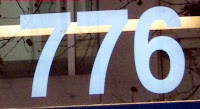In the film Bruce Almighty, God contacts Bruce, via pager, using an actual phone number rather than a number in the standard fictional 555 telephone exchange: 776-2323.

## Monday, August 23, 2010

### 400

400 = 24 x 52.

400 = 202, a perfect square.

400 = 70 + 71 + 72 + 73. Hence, 400 is 1111 in base 7.

400 has a representation as a sum of two squares: 400 = 122 + 162.

A full circle is 360 degrees or 400 grads.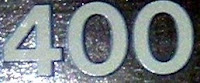Years divisible by 400 are leap years, following adoption of the current Gregorian calendar.

Source: Numeropedia

## Friday, August 20, 2010

### 6989

6989 = 29 x 241.

6989 has two representations as a sum of two squares: 6989 = 102 + 832 = 502+ 672.

6989 is the hypotenuse of two primitive Pythagorean triples: 69892 = 16602 + 67892 = 19892 + 67002.

6989 is a divisor of 308 - 1.

6989 has the property that the concatenation of its prime factors in increasing order is a square: 29241 = 1712.6989 "Wightwick Hall," currently being restored, was the second last steam locomotive to be commissioned by the Great Western Railway Company before British Railways took over.

## Thursday, August 19, 2010

### 676

676 = 22 x 132.

676 = 262, a perfect square.

676 is the smallest palindromic square whose square root is not palindromic.

676 has a representation as a sum of two squares: 676 = 102 + 242.

676 is a divisor of 994 - 1.

676 is 1010100100 in base 2 (binary).676 Melitta is an asteroid discovered in 1909.

## Wednesday, August 18, 2010

### 81

81 = 34, a perfect fourth power.

81 is the only integer other than 1 that is the square of the sum of its digits.

81 is a centered octagonal number.

81 = 1 + 3 + 5 + 7 + 9 + 11 + 13 + 15 + 17 (the first nine odd numbers).The chemical element thallium has the atomic number 81.

## Tuesday, August 17, 2010

### 368

368 = 24 x 23.

368 is the number of ways to tile a 4 x 15 rectangle with pentominoes.

368 is the sum of three positive cubes: 368 = 63 + 53 + 33.

368 is a number that cannot be written as a sum of three squares.

368 is a Leyland number.In 2009, The Princeton Review published the guide: The Best 368 Colleges.

## Monday, August 16, 2010

### 282

282 = 2 x 3 x 47.

282 is the smallest multidigit palindrome sandwiched between twin primes: 281 and 283.

282 is 2112 in base 5 and 343 in base 9.

282 is the number of planar partitions of 9.The code of Hammurabi contained 282 laws.

## Friday, August 13, 2010

### 845

845 = 5 x 132.

845 is 1101001101 in base 2 (binary) and 31031 in base 4; it is 1515 in base 8 and 500 in base 13.

845 has three representations as a sum of two squares: 845 = 22 + 292 = 132 + 262 = 192 + 222.

845 is the hypotenuse of two primitive Pythagorean triples: 8452 = 1162 + 8372 = 1232 + 8362.

845 is a number n for which 8n + 1 and 8n + 3 are twin primes: 8 x 845 + 1 = 6761 and 8 x 845 + 3 = 6763.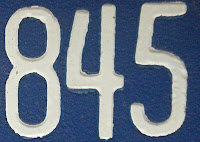845 Naema is an asteroid discovered in 1916.

## Thursday, August 12, 2010

### 259

259 = 7 x 37.

259 is 1111 in base 6.

259 is a 14-gonal number.

259 is a lucky number.

259 is the solution to the postage-stamp problem for three denominations and 13 stamps.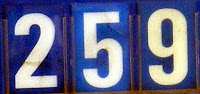259 hectares is equivalent to 1 square mile.

## Wednesday, August 11, 2010

### 332

332 = 22 x 83.

332 is the number of 2-connected graphs with 7 vertices.

332 is 110022 in base 3; it is 2312 in base 5 and 1312 in base 6.

332 is a number n such that the sum of the first n primes is a prime.

332 is the number of primes less than 10,000 having at least one digit 6.Tiger 332 is a German Panzer tank from World War II on display at the Patton Museum of Cavalry and Armor in Fort Knox, Kentucky.